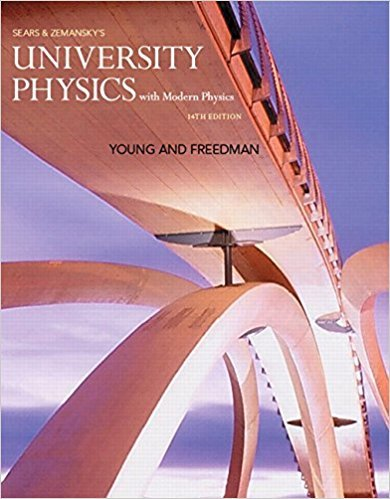×
×

# (a) If the momentum of a single point object is equal toISBN: 9780321973610 228

## Solution for problem Q8.6 Chapter 8

University Physics with Modern Physics (1) | 14th Edition

• Textbook Solutions
• 2901 Step-by-step solutions solved by professors and subject experts
• Get 24/7 help from StudySoup virtual teaching assistantsUniversity Physics with Modern Physics (1) | 14th Edition

4 5 0 291 Reviews
16
4
Problem Q8.6

(a) If the momentum of a single point object is equal to zero, must the objects kinetic energy also be zero? (b) If the momentum of a pair of point objects is equal to zero, must the kinetic energy of those objects also be zero? (c) If the kinetic energy of a pair of point objects is equal to zero, must the momentum of those objects also be zero? Explain your reasoning in each case.

Step-by-Step Solution:
Step 1 of 3

Chapter4:Two-DimensionalKinematics Ittakes1numbertospecifyapositionona1-dimensionalline,2numbers fora2-dimensionalspace,and3numbersfora3-dimensionalspace. Tocreateacoordinatesystemin2-dimensionstakeapointattheoriginand definetwoperpendiculardirectionstoorientthesystem. *curlynotaionwithmagnitudeanddirectionasthetwonumber s;itispolar planesystem...

Step 2 of 3

Step 3 of 3

##### ISBN: 9780321973610

The full step-by-step solution to problem: Q8.6 from chapter: 8 was answered by , our top Physics solution expert on 01/09/18, 07:46PM. This full solution covers the following key subjects: . This expansive textbook survival guide covers 44 chapters, and 4574 solutions. Since the solution to Q8.6 from 8 chapter was answered, more than 241 students have viewed the full step-by-step answer. This textbook survival guide was created for the textbook: University Physics with Modern Physics (1), edition: 14. The answer to “(a) If the momentum of a single point object is equal to zero, must the objects kinetic energy also be zero? (b) If the momentum of a pair of point objects is equal to zero, must the kinetic energy of those objects also be zero? (c) If the kinetic energy of a pair of point objects is equal to zero, must the momentum of those objects also be zero? Explain your reasoning in each case.” is broken down into a number of easy to follow steps, and 75 words. University Physics with Modern Physics (1) was written by and is associated to the ISBN: 9780321973610.

Unlock Textbook Solution